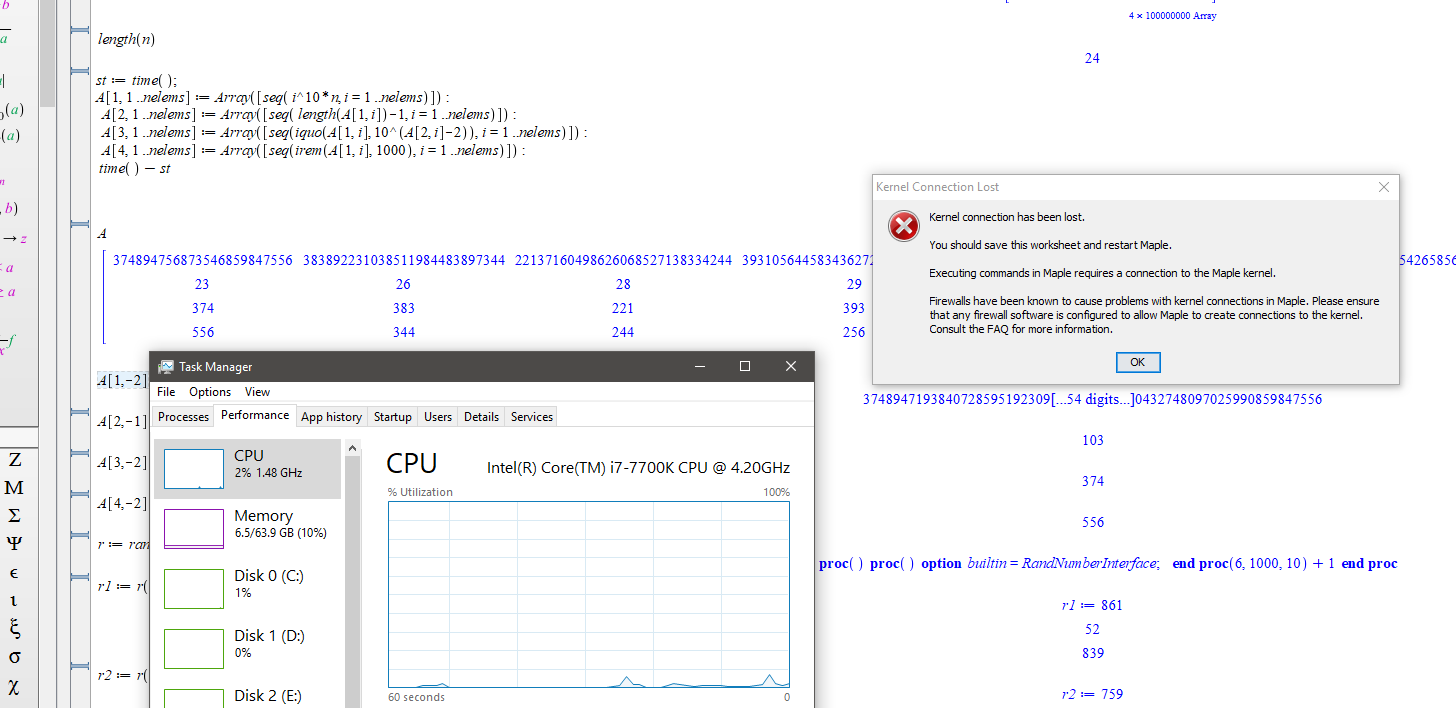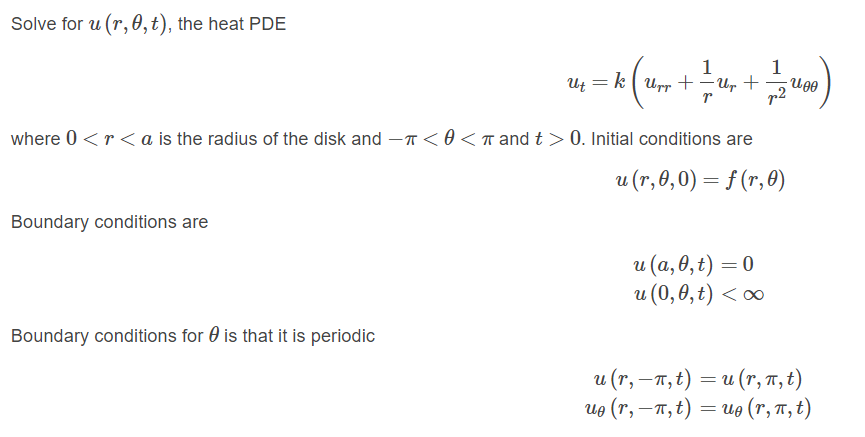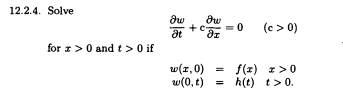## Game of Thrones and Graph Theory

by: Maple 2019

While googling around for Season 8 spoilers, I found data sets that can be used to create a character interaction network for the books in the A Song of Ice and Fire series, and the TV show they inspired, Game of Thrones.

The data sets are the work of Dr Andrew Beveridge, an associate professor at Macalaster College (check out his Network of Thrones blog).

You can create an undirected, weighted graph using this data and Maple's GraphTheory package.

Then, you can ask yourself really pressing questions like

• Who is the most influential person in Westeros? How has their influence changed over each season (or indeed, book)?
• How are Eddard Stark and Randyll Tarly connected?
• What do eigenvectors have to do with the battle for the Iron Throne, anyway?

These two applications (one for the TV show, and another for the novels) have the answers, and more.

The graphs for the books tend to be more interesting than those for the TV show, simply because of the far broader range of characters and the intricacy of the interweaving plot lines.

Let’s look at some of the results.

This a small section of the character interaction network for the first book in the A Song of Ice and Fire series (this is the entire visualization - it's big, simply because of the shear number of characters)

The graph was generated by GraphTheory:-DrawGraph (with method = spring, which models the graph as a system of protons repelling each other, connected by springs).

The highlighted vertices are the most influential characters, as determined by their Eigenvector centrality (more on this later).The importance of a vertex can be described by its centrality, of which there are several variants.

Eigenvector centrality, for example, is the dominant eigenvector of the adjacency matrix, and uses the number and importance of neighboring vertices to quantify influence.

This plot shows the 15 most influential characters in Season 7 of the TV show Game of Thrones. Jon Snow is the clear leader.Here’s how the Eigenvector centrality of several characters change over the books in the A Song of Ice and Fire series.A clique is a group of vertices that are all connected to every other vertex in the group. Here’s the largest clique in Season 7 of the TV show.Game of Thrones has certainly motivated me to learn more about graph theory (yes, seriously, it has). It's such a wide, open field with many interesting real-world applications.

Enjoy tinkering!

## How to change zoom factor in worksheet to a value ...

I googled, read help and not able to find how to do this.

I want to change the zoom in worksheet to any value I want, and not just 100%,150%,75%, etc.... which are the values that are listed in View->Zoom factor.

Is there a place one can type in the zoom value they want? Say 110% or any other number?

windows 10.

Thank you

## How to parse Maple string that contains more than ...

I read a string from file which contains a sequence of Maple commands, all in one string. The commands are separated by ";" in the string. I need to then evaluate all the commands in the string as if I typed them one after the other.

Here is an example

```data:="x:='x';y:='y';sol:=dsolve(diff(y(x),x)=1,y(x));"; #read from database
parse(data);```

Warning, extra characters at end of parsed string
x := 'x'

I want the result to parse to be as if I typed

```x:='x';
y:='y';
sol:=dsolve(diff(y(x),x)=1,y(x));```

I looked at help in parse page, but I do not see what I need to do. It says that

If the option 'statement' is specified, the string must consist of exactly one Maple statement (which includes expressions). The statement is parsed and evaluated, and the result is returned.

But I am not using the statment option. I also tried using this option, no change, same problem.

Is there a way to prase such a string?

Thanks

## column name missing in FetchRow for Database[SQLit...

Is this documenation wrong?

https://www.maplesoft.com/support/help/maple/view.aspx?path=Database%2fSQLite%2fFetchRow

It says that calling sequence is

FetchRow( statement, column, valuetype  )

But below it only shows

 statement - prepared statement obtained using Prepare command valuetype - type of the output data, default is "auto"

With no column anywhere.  And none of examples show column in them.

And when I tried it by adding a column name that I know exist in my sqlite3 database, Maple gave an error that no such parameter allowed.

Does FetchRow support column name or is the above just a documenation error?

Maple 2019

## Odditity in Maple 2019...

I have noticed a few times now with Maple 2019. It looses kernel connection when it is sitting there idly. This time I observed it. Had saved a document after an intensive calculation. The memory used was about 30Gig. shortly after saving the cpu fan was running hard. I checked task manager and cpu was cycling to 100%, it was mserever. Then the memory usage droped to about 6gig and message as shown. During this time Maple screen down in the LH corner displayed "Ready", so it didn't think it was doing anything.## Can seq or Seq be applied to fill our a 2 d Array?...

My code below works but I an looking to speed it up to apply to large arrays. I have large integers and I want to store the exponent and first and last few digits.

```nelems := 10;  ~ takes about 50mins when n=100,000,000
n := 374894756873546859847556;
op(n);
A := Array(1 .. 4, 1 .. nelems);
length(n);
st := time();
for i to nelems do
A[1, i] := i^10*n;
A[2, i] := length(A[1, i]) - 1;
b := convert(A[1, i], string);
A[3, i] := parse(b[1 .. 3]);
A[4, i] := parse(b[-3 .. -1]);
end do;
time() - st;
A;
A[1, -2];
A[2, -2];
A[3, -2];
A[4, -2];
```

## simplify equation ...

Hello,

How I can take variation from left-hand side of  5, and reach to right-hand side of  5. After by using integral by part obtained  7?

Thank you## can Maple 2019 solve the heat PDE inside disk when...

Maple pdsolve supports periodic boundary conditions. So I was hoping it will be able to solve the heat PDE inside disk with periodic boundary conditions. But I am not able to make it work.

Is there a trick to make Maple solve this, is there something I need to add or adjust something else? or it is just the functionality is not currently implemented?This is what I tried

```restart;

pde := diff(u(r,theta,t),t)=diff(u(r,theta,t),r\$2) + 1/r*diff(u(r,theta,t),r)+1/r^2*diff(u(r,theta,t),theta\$2);
bc1 := u(a,theta,t)=0;
bc2 := eval(diff(u(r,theta,t),theta),theta=-Pi)=eval(diff(u(r,theta,t),theta),theta=Pi);
bc3 := u(r,-Pi,t)=u(r,Pi,t);
ic  := u(r,theta,0)=f(r,theta);
sol := pdsolve([pde, bc1,bc2,bc3, ic], u(r, theta, t), HINT = boundedseries(r = 0)) assuming a>0,r>0
```

I solved this analytically by hand using standard separation of variables method. The issue of telling Maple the solution is bounded at center of disk, I assume is being handled automatically by the HINT=boundedseries(r = 0).

If I remove the hint, it also does not solve it.

Maple 2019, Physics package 338

## how to obtain this solution for wave PDE in 1D in ...